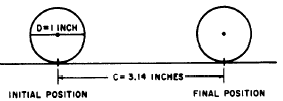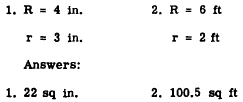Formulas for Circumference and AreaCustom SearchFormulas for Circumference and Area The formula for the circumference of a circle is based on the relationship between the flat surface. (See fig. 17-19.) The distance from the initial position to the final position of the disk in figure 17-19 is approximately 3.14 times as long as the diameter letter pi. Thus we have the following equations:Figure 17-18.-Arc, chord, segment, and sector.Figure 17-19.-Measuring the circumference of a circle. This formula states that the circumference of a circle is n times the diameter. Notice that it could be written as C = 2r � p or C = 2pr since the diameter d is the same as 2r (twice the radius), Although the value of p is not exactly equal to any of the numerical expressions which are sometimes used for it, the ratio is very close to 3.14. If extreme accuracy is required, 3.1416 is used as an approximate value of p. Many calculations involving p are satisfactory if the fraction 22/7 is used as the value of p. Practice problems. Calculate the circumference of each of the following circles, using 22/7 as the value of p: 1. Radius = 21 in.  2. Diameter = 7.28 in.  3. Radius = 14 ft 4. Diameter = 2.8 yd Answers: 1. 132 in.  2. 22.88 in.  3. 88 ft 4. 8.8 yd AREA.-The area of a circle is found formula is written as follows: A = pr2 EXAMPLE: Find the area of a circle whose diameter is 4 ft, using 3.14 as the value of p. SOLUTION: The radius is one-half the diameter. Therefore,Practice problems. Find the area of each of the following circles, using 3.14 as the value of p. 1. Radius = 7 in.  2. Diameter = 42 mi  3. Diameter = 2.8 ft 4. Radius = 14 yd Answers: 1. A = 154 sq in. 2. A = 1,385 sq ml 3. 6.15 sq ft 4. 615 sq yd Concentric Circles Circles which have a common center are said to CONCENTRIC. (See fig. 17-20.) The area bf the ring between the concentric circles in figure 17-20 is calculated as follows:Figure 17-20.-Concentric circles.Notice that the last expression is the difference of two squares. Factoring, we have A = p(R + r)(R - r) Therefore, the area of a ring between two circles is found by p times the product of the sum and difference of their radii. Practice problems. Find the areas of the rings the following concentric circles:Integrated Publishing, Inc. - A (SDVOSB) Service Disabled Veteran Owned Small Business